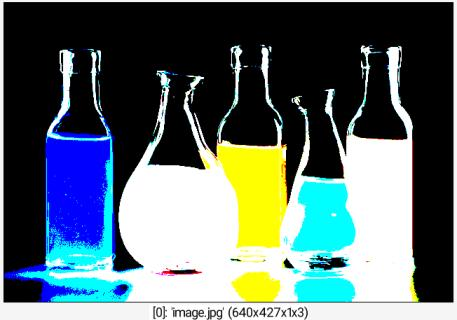Reference

 Table of Contents  ▸  List of Commands  ▸  Mathematical Operators  ▸  le ◀  gt    |    lt  ▶

# le

Built-in command

## Arguments:

• value[%]    or
• [image]    or
• 'formula'    or
• (no arg)

## Description:

Compute the boolean 'less or equal than' of selected images with specified value, image or mathematical expression, or compute the boolean 'less or equal than' of selected images.

(equivalent to shortcut command <=).

## Examples of use:

### • Example #1

\$ gmic image.jpg le {ia}Command: image.jpg le {ia}

### • Example #2

\$ gmic image.jpg +mirror x leCommand: image.jpg +mirror x le